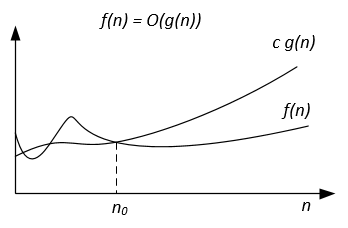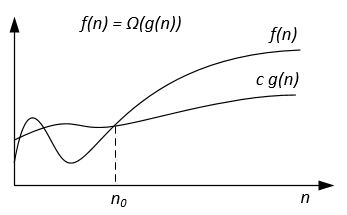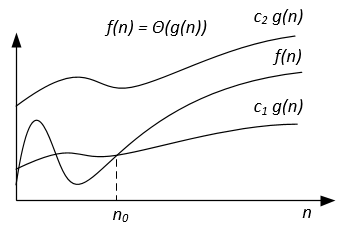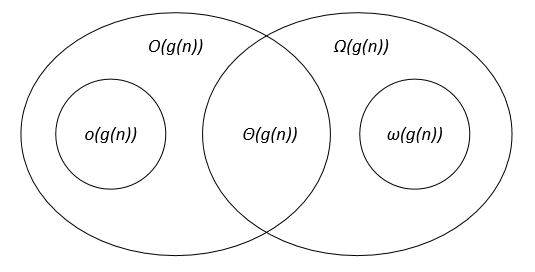# Comparison of the asymptotic notations

suggest change

Let `f(n)` and `g(n)` be two functions defined on the set of the positive real numbers, `c, c1, c2, n0` are positive real constants.

Notation | f(n) = O(g(n)) | f(n) = Ω(g(n)) | f(n) = Θ(g(n)) | f(n) = o(g(n)) | f(n) = ω(g(n)) | —— | —— | —— | —— | —— | —— | Formal definition | `∃ c > 0, ∃ n0 > 0 : ∀ n ≥ n0, 0 ≤ f(n) ≤ c g(n)` | `∃ c > 0, ∃ n0 > 0 : ∀ n ≥ n0, 0 ≤ c g(n) ≤ f(n)` | `∃ c1, c2 > 0, ∃ n0 > 0 : ∀ n ≥ n0, 0 ≤ c1 g(n) ≤ f(n) ≤ c2 g(n)` | `∀ c > 0, ∃ n0 > 0 : ∀ n ≥ n0, 0 ≤ f(n) < c g(n)` | `∀ c > 0, ∃ n0 > 0 : ∀ n ≥ n0, 0 ≤ c g(n) < f(n)` | Analogy between the asymptotic comparison of `f, g` and real numbers `a, b` | `a ≤ b` | `a ≥ b` | `a = b` | `a < b` | `a > b` | Example | `7n + 10 = O(n^2 + n - 9)` | `n^3 - 34 = Ω(10n^2 - 7n + 1)` | `1/2 n^2 - 7n = Θ(n^2)` | `5n^2 = o(n^3)` | `7n^2 = ω(n)` | Graphic interpretation | | | | | |The asymptotic notations can be represented on a Venn diagram as follows: